## Posts

Showing posts from March 6, 2022

### Measuring the Speed of Light: Michelson's MethodIn 1926, Albert Michelson, the German-born American physicist, came up with a method to measure the speed of light to a high degree of accuracy despite the technology not being advanced enough at that time. In recognition of his achievement in the field of science, he was awarded the Nobel Prize for physics in 1907. He managed to mount an octagonal-shaped prism on Mount Wilson  and a combination of a concave mirror and a plane mirror on another mountain peak, 35km away in such a way that both were in line of sight.     The prism was mounted on a shaft attached to a variable motor the speed of which Michelson could change. A beam of light was then arranged to hit the revolving octagonal prism. The beam, reflected at a side of the prism, then went and it the concave mirror and the plane mirror respectively, before coming back to hit the prism again.  Michelson managed to control the speed of the rotation until he could see the reflected beam through a telescope.   He found out w

### Python Algorithms for Computer Science - Euclid Algorithm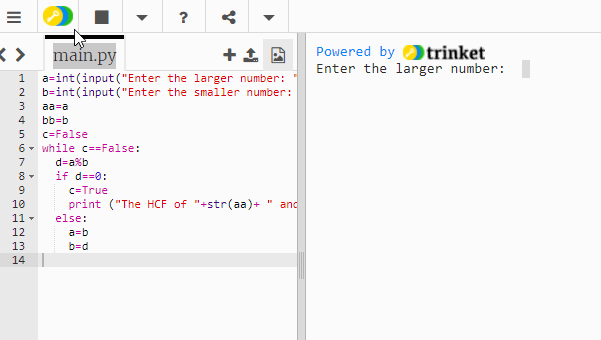Euclid's Algorithm is one of the oldest algorithms known to  mankind. It was found in Euclid's Elements, something that dates back to 300 B.C. When Euclid came up with the algorithm, mathematics was not known as it is today; in fact, he used geometrical methods in his discovery, as algebra was not known at that time, as a branch of mathematics. Euclid's algorithm is used to find the HCF - Highest Common Factor - or GCD - Greatest Common Divider - of two numbers. The following image shows the steps in the algorithm: This is the flowchart for the algorithm: You can practise the algorithm with the following Python code snippet: If you want to practise the JavaScript version, please follow this link .

### Instantaneous Velocity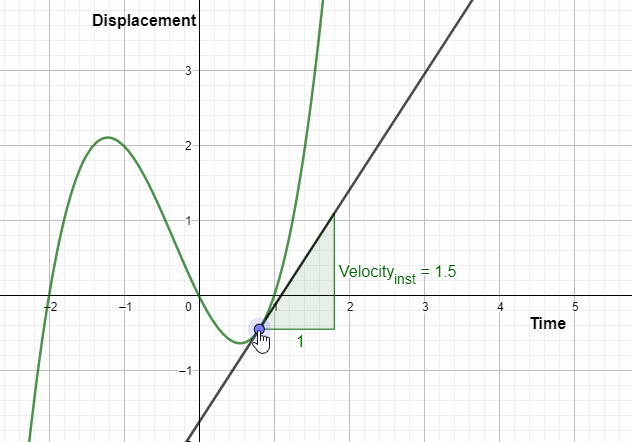The instantaneous velocity of an object can be found by the gradient of the displacement/time graph at a given time.  E.g. In the above graph, which you can practise interactively below, the gradient, when the time, t = 1s, is as follows: v = (3 - 0)/(1 - 0) = 3 m/s You can practise the calculations interactively here; please move point:

### Histograms Simulator: GCSE, IGCSE, AS, A Level, IB, GCE O L and A L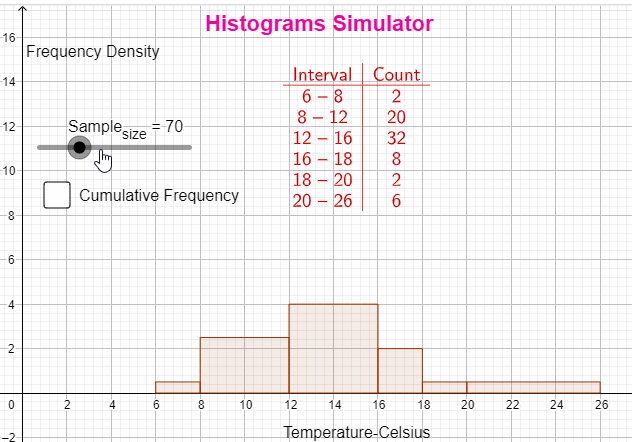A histogram represents continuous grouped data as a running total in each group. The area of a each bar, as opposed to the height of each bar, represents the frequency of each group or bar. The greater the area, the greater the frequency. Since classes are not of equal width, as this is the case in a bar chart,  the y-axis represents the frequency density, instead of frequency of a given set of data. frequency density = frequency / class width You can practise histograms with the following applet:

### Refraction of Light - simulator for GCSE, IGCSE, A Level, IB, GCE O Level, GCE A Level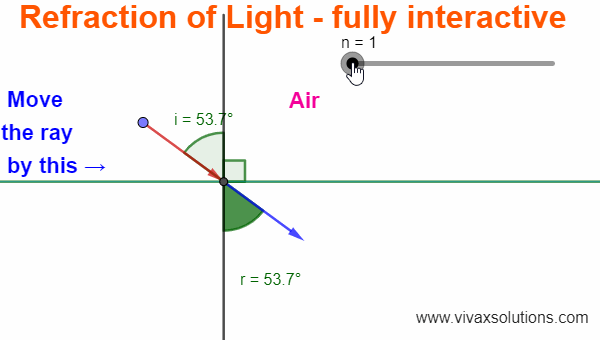Refraction When light moves from a medium to another medium, its speed and the direction changes. This is called refraction of light. Snell's Law When light refracts, the ratio of the sine of the angle of incidence to the ratio of the sine of the angle of refraction remains the same for any two given media. Equation of Refraction n 1 sin i 1 = n 2 sin i 2   E.g. 1 A ray of light goes from air to glass at an angle of 60 0 to the surface. If the refractive indices of air and glass are 1 and 1.5 respectively, find the angle of refraction and determine the nature of refraction. n 1 = 1 n 2 = 1.5 i = 30 r = ? 1 sin 30 = 1.5 sin r sin r = 0.5/1.5 = 1/3 r = sin -1 (1/3) r = 19.6 0 The ray bends towards the normal; hence, the latter is a denser medium. E.g. 2 A ray of light goes from water to air at an angle of 50 0 to the surface. If the refractive indices of air and water are 1 and 1.3 respectively, find the angle of refraction and determine the nature of refraction. n 1 =

### Frequency Amplitude and Wavelength of a Transverse Wave - simulation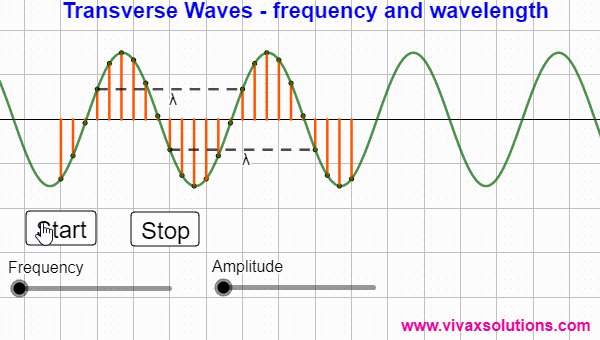Waves A wave carries energy from a point to another point in a medium / vacuum without the movement of the particles along with it. In the above animation, the wave moves from the left to the right; the particles, however, just vibrate vertically without moving along with the wave. Transverse Waves If the direction of the wave is perpendicular to the vibration of particles, it is called a transverse wave. E.g. Water waves, radio waves, microwaves Amplitude The maximum displacement of a particle in a wave is called amplitude. Intensity / loudness The intensity / loudness of a wave is proportional to the square of the amplitude. The greater the amplitude, the greater the intensity / loudness. Amplifier This is what an amplifier does: it increases the amplitude of an input signal, which in turn leads to the increased loudness or intensity. Frequency  - f The number of cycles of a wave that passes through a point in a unit time is called frequency. Wavelength  - λ The distance between